# Theorem 1: The Sine LawStatement: In any triangle the sides are proportional to the Sine of the opposite angles.
﻿In other words, in any ΔABCProof:
↪ Let ABC be a triangle with a = BC, b = CA and c = AB, then three cases are possible. The angle C is either acute or right or obtuse angle.↪ Draw AD perpendicular to BC ( produce BC if necessary )

In ΔABC, SinB =for all figures

or, AD = cSinB → (i)

Also in
ΔACD,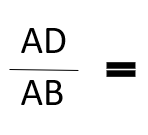1 { SinC in fig (i), Sin90 = SinC in fig (ii) and Sin(π-C) = SinC in fig (iii) }

Therefore for all the figures,
SinC =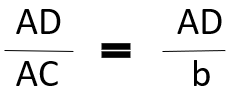↣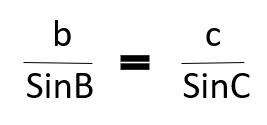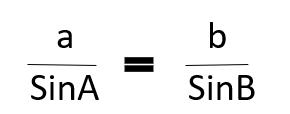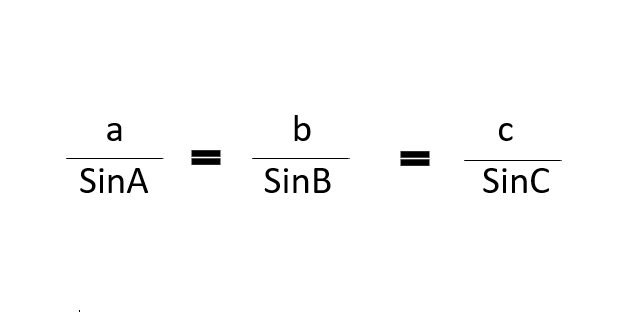Proved.